# Ensemble Modeling

In the world of analytics,modeling is a general term used to refer to the use of data mining (machine learning) methods to develop predictions. If you want to know what ad a particular user is more likely to click on, or which customers are likely to leave you for a competitor, you develop a predictive model.

There are a lot of models to choose from: Regression, Decision Trees, K Nearest Neighbor, Neural Nets, etc. They all will provide you with a prediction, but some will do better than others depending on the data you are working with. While there are certain tricks and tweaks one can do to improve the accuracy of these models, it never hurts to remember the fact that there is wisdom to be found in the masses.

### The Jelly Bean Jar

I am sure everyone has come across some version of this in their life: you are at a fair or school fund raising event and someone has a large see-through jar full of jelly beans (or marbles or nickles). Next to the jar are some slips of paper with the instructions to “Guess the number of jelly beans in the jar you win!”

An interesting thing about this game, and you can try this out for yourself, is that given a reasonable number of participants, more often than not, the average guess of the group will perform better than the best individual guesser. Or in other words, imagine there are 200 jelly beans in the jar and the best guesser (the winner) guesses 215. More often than not, the average of all the guesses might be something like 210 or 190. The group cancels out their over and under guessing, resulting in a better answer than anyone individual.

### How Do We Get the Average in Models?

There are countless ways to do it, and researchers are constantly trying new approaches to get that extra 2% improvement over the last model. For ease of understanding though, I am going to focus on 2 very popular methods of ensemble modeling : Random Forests & Boosted Trees.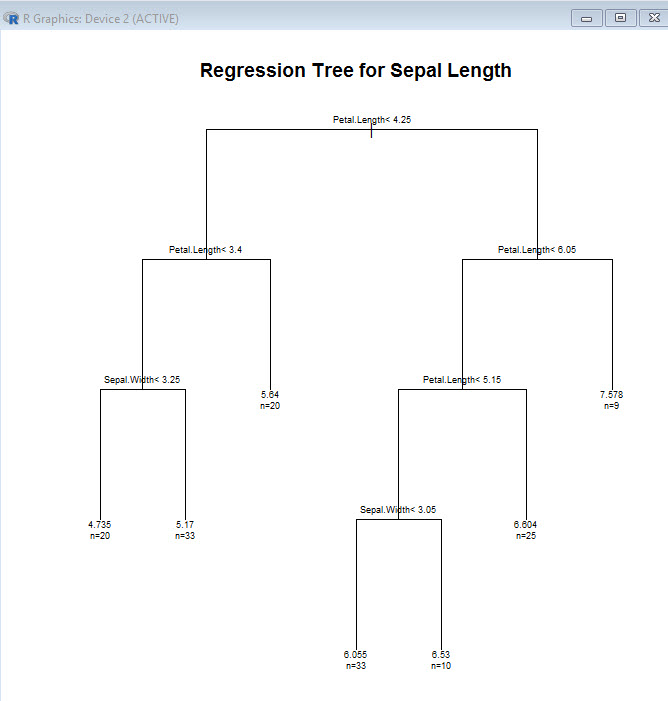Random Forests:

Imagine you have a data set containing 50,000 records. We will start by randomly selecting 1000 records and creating a decision tree from those records. We will then put the records back into the data set and draw another 1000 records, creating another decision tree. The process is repeated over and over again for a predefined number of iterations (each time the data used is returned to the pool where it could possibly be picked again).

After all the sample decision trees have been created (let’s say we created 500 for the sake of argument), the model then takes the mean or average of all the models if you are looking at a regression or the mode of all the models if you are dealing with a classification.

For those unfamiliar with the terminology, a regression model looks for a numeric value as the answer. It could be the selling price of a house, a person’s weight, the price of a stock, etc. While a classification looks for classifying answers: yes or no, large – medium – small, fast or slow, etc.

Boosted Trees:

Another popular method of ensemble modeling is known as boosted trees. In this method, a simple (poor learner) model tree is created – usually 3-5 splits maybe. Then another small tree (3-5 splits) is built by using incorrect predictions for the first tree. This is repeated multiple times (say 50 in this example), building layers of trees, each one getting a little bit better than the one before it. All the layers are combined to make the final predictive model.

### Oversimplified?

Now I know this may be an oversimplified explanation, and I will create some tutorials on actually building ensemble models, but sometimes I think just getting a feel for the concept is important.

So are ensemble models always the best? Not necessarily.

One thing you will learn when it comes to modeling is that no one method is the best. Each has their own strengths. The more complex the model, the longer it takes to run, so sometimes you will find speed outweighs desire for the added 2% accuracy bump. The secret is to be familiar with the different models, and to try them out in different scenarios. You will find that choosing the right model can be as much of an art as a science.

# R: K-Means Clustering- Deciding how many clusters

In a previous lesson I showed you how to do a K-means cluster in R. You can visit that lesson here: R: K-Means Clustering.

Now in that lesson I choose 3 clusters. I did that because I was the one who made up the data, so I knew 3 clusters would work well. In the real world it doesn’t work that way. Choosing the right number of clusters is one of the trickier parts of performing a k-means cluster.

If you go over to Michael Grogan’s site, you will see he has a great method for figuring out how many clusters to choose. http://www.michaeljgrogan.com/k-means-clustering-example-stock-returns-dividends/

````wss <- (nrow(sample_stocks)-1)*sum(apply(sample_stocks,2,var))`
`for (i in 2:20) wss[i] <- sum(kmeans(sample_stocks,`
`centers=i)\$withinss)`
`plot(1:20, wss, type="b", xlab="Number of Clusters",`
`ylab="Within groups sum of squares")````

If you understand the code above, then great. That is a great solution for choosing the number of clusters. If, however, you are not 100% sure what is going on above, keep reading. I’ll walk you through it.

## K-Means Clustering

We need to start by getting a better understanding of what k-means clustering means. Consider this simplified explanation of clustering.

The way is works is each of the rows our data are placed into a vector.These vectors are then plotted out in space. Centroids (the yellow stars in the picture below) are chosen at random. The plotted vectors are then placed into clusters based on which centroid they are closest to.So how do you measure how good your clusters fit. (Do you need more clusters? Less clusters)? One popular metrics is the Within cluster sum of squares. R provides this as kmeans\$withinss. What this means is the distance the vectors in each cluster are from their respected centroid.

The goal is to get the is to get this number as small as possible. One approach to handling this is to run your kmeans clustering multiple times, raising the number of the clusters each time. Then you compare the withinss each time, stopping when the rate of improvement drops off. The goal is to find a low withinss while still keeping the number of clusters low.This is, in effect, what Michael Grogan has done above.

## Break down the code

Okay, now lets break down Mr. Grogan’s code and see what he is doing.

````wss <- (nrow(sample_stocks)-1)*sum(apply(sample_stocks,2,var))`
`for (i in 2:20) wss[i] <- sum(kmeans(sample_stocks,`
`centers=i)\$withinss)`
`plot(1:20, wss, type="b", xlab="Number of Clusters",`
`ylab="Within groups sum of squares")````

The first line of code is a little tricky. Let’s break it down.

`wss <- (nrow(sample_stocks)-1)*sum(apply(sample_stocks,2,var))`

sample_stocks – the data set

wss <-  – This simply assigns a value to a variable called wss

(nrow(sample_stocks)-1)  – the number of rows (nrow) in sample_stocks – 1. So if there are 100 rows in the data set, then this will return 99

sum(apply(sample_stocks,2,var)) – let’s break this down deeper and focus on the apply() function. apply() is kind of like a list comprehension in Python. Here is how the syntax works.

apply(data, (1=rows, 2=columns), function you are passing the data through)

So, let’s create a small array and play with this function. It makes more sense when you see it in action.

``` tt <- array(1:20, dim=c(10,2)) # create array with data 1 -20,
#10 rows, 2 columns
> tt
[,1] [,2]
[1,] 1 11
[2,] 2 12
[3,] 3 13
[4,] 4 14
[5,] 5 15
[6,] 6 16
[7,] 7 17
[8,] 8 18
[9,] 9 19
[10,] 10 20```

Now lets try running this through apply.

```> apply(tt, 2, mean)
 5.5 15.5```

Apply took the mean of each column. Had I used 1 as the second argument, it would have taken the mean of each row.

```> apply(tt, 1, mean)
 6 7 8 9 10 11 12 13 14 15```

Also, keep in mine, you can create your own functions to be used in apply

```apply(tt,2, function(x) x+5)
[,1] [,2]
[1,] 6 16
[2,] 7 17
[3,] 8 18
[4,] 9 19
[5,] 10 20
[6,] 11 21
[7,] 12 22
[8,] 13 23
[9,] 14 24
[10,] 15 25
```

So, what is Mr. Grogan’s doing with his apply function? apply(sample_stocks,2,var) – He is taking the variance of each column his data set.

``` apply(tt,2,var)
 9.166667 9.166667```

And by summing it: sum(apply(sample_stocks,2,var)) – he is simply adding the two values together.

``` sum(apply(tt,2,var))
 18.33333```

So, the entire first line of code using our data is:

wss <- (nrow(tt)-1)*sum(apply(tt,2,var))

wss <- (10-1) * (18.333)

```wss <- (nrow(tt)-1)*sum(apply(tt,2,var))
> wss
 165```

What this number is effectively is the within sum of squares for a data set that has only one cluster

### Next section of code

Next we will tackle the next two lines of code.

````for (i in 2:20) wss[i] <- sum(kmeans(sample_stocks,`
`centers=i)\$withinss)````

The first part is a for loop and should be simple enough. Note he doesn’t use {} to denote the inside of his loop. You can do this when your for loop is a single line, but I am going to use the {}’s anyway, as I think it makes the code a bit neater.

for (i in 2:20)  — a for loop iterating from 2 -20

for (i in 2:20) {

wss[i] <- }  – we are going to assign more values to the vector wss starting at 2 and working our way down to 20.

Remember, a single value variable in R is actually a single value vector.

```c <- 5
> c
 5
> c <- 7
> c
 5 7```

Okay, so now to the trickier code. sum(kmeans(sample_stocks, centers = i)\$withinss)

What he is doing is running a kmeans cluster for the data one time each for each value of centers (number of centroids we want) from 2 to 20 and reading the \$withinss from each run. Finally it sums all the withinss up (you will have 1 withinss for every cluster you create – number of centers)

### Plot the results

The last part of the code is plotting the results

````plot(1:20, wss, type="b", xlab="Number of Clusters",`
`ylab="Within groups sum of squares")````

plot (x, y, type= type of graph, xlab = label for x axis, ylab= label for y axis

## Let’s try it with our data

``` myData <- read.csv('cluster.csv')
StudentId TestA TestB
1 2355645.1 134 24
2 8718152.6 155 32
3 8303333.6 130 25
4 6352972.5 185 86
5 3381543.2 153 95
6 817332.4 153 81
> myData <- myData [,2:3] # get rid of StudentId column
TestA TestB
1 134 24
2 155 32
3 130 25
4 185 86
5 153 95
6 153 81```

Now lets feed this through Mr. Grogan’s code

````wss <- (nrow(myData)-1)*sum(apply(myData,2,var))`
```for (i in 2:20) {
wss[i] <- sum(kmeans(myData,```
```          centers=i)\$withinss)
```         }
`plot(1:20, wss, type="b", xlab="Number of Clusters",`
`ylab="Within groups sum of squares")````

Here is our output ( a scree plot for you math junkies out there)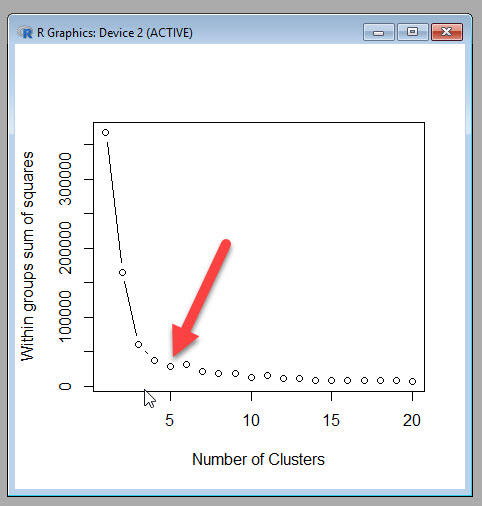Now Mr. Grogan’s plot a nice dramatic drop off, which is unfortunately not how most real world data I have seen works. I am going to chose 5 as my cut off point, because while the withinss does continue to decrease, it doesn’t seem to do so at a rate great enough to accept the added complexity of more clusters.

If you want to see how 5 clusters looks next to the three I had originally created, you can run the following code.

```myCluster <- kmeans(myData,5, nstart = 20)
myData\$cluster <- as.factor(myCluster\$cluster)
ggplot(myData, aes(TestA, TestB, color = cluster))
+ geom_point()```

5 Clusters3 Clusters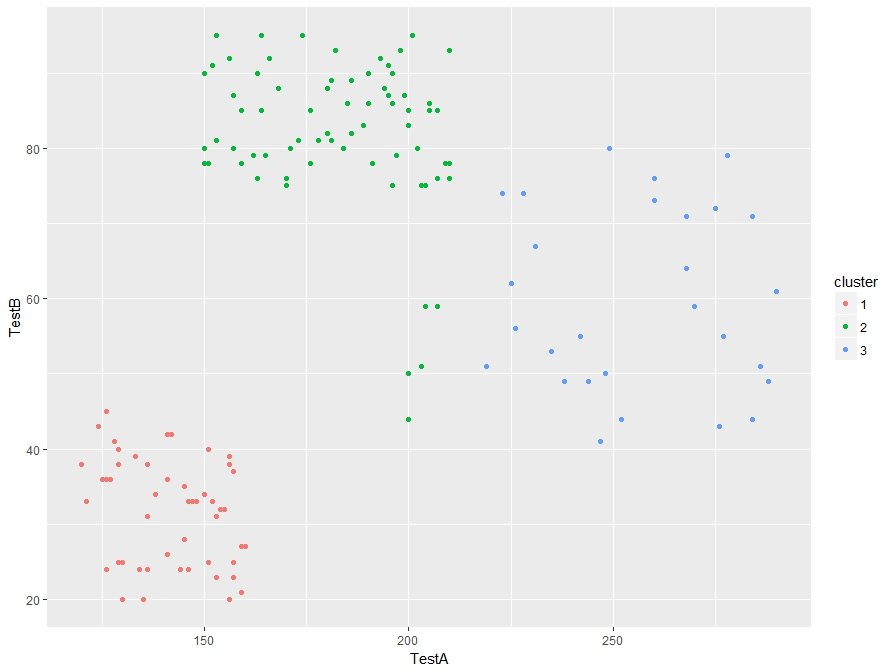I see some improvement in the 5 cluster model. So Michael Grogan’s trick for finding the number of clusters works.

# R: K-Means Clustering

Note: This is an introductory lesson with a made up data set. After you are finished with this tutorial, if you want to see a nice real world example, head on over to Michael Grogan’s website:

http://www.michaeljgrogan.com/k-means-clustering-example-stock-returns-dividends/

K Means Cluster will be our introduction to Unsupervised Machine Learning. What is Unsupervised Machine Learning exactly? Well, the simplest explanation I can offer is that unlike supervised where our data set contains a result, unsupervised does not.

Think of a simple regression where I have the square footage and selling prices (result) of 100 houses. Taking that data, I can easily create a prediction model that will predict the selling price of a house based off of square footage. – This is supervised machine learning

Now, take a data set containing 100 houses with the following data: square footage, house style, garage/no garage, but no selling price. We can’t create a prediction model since we have no knowledge of prices, but we can group the houses together based on commonalities. These groupings (clusters) can be used to gain knowledge of your data set.

I think seeing it in action will help.

Here is the data set: cluster

The data we will be looking at test results for 149 students.The task at hand is to group the students into 3 groups based on the test results. Now one thing any teacher will let you know is that some kids perform well in one subject and perhaps not so well in another. So we can’t simply group them on the score performance on one test. And when you are dealing with real world data, you might be looking at 20 -100 test/quiz scores per student.

So what we are going to do is let the computer decide how to group (or cluster) them.

To do so, we are going to be using K-means clustering. K-means clustering works by choosing random points (centroids). It then groups the data points around the centroids based which centroid the points are closest to.

## Let’s get started

```st <- read.csv(file.choose())

our dataNow let’s run the data through a Kmeans() algorithm

First, we are only going to want to focus on columns 2 and 3 in the data set since column 1 (studentID) is basically a label and provides no value in prediction.

To do this, we subset the data: st[,2:3] – which means I want all row ([,) and columns 2-3 (2:3])Now the code to make our clusters

```stCl <- kmeans(st[, 2:3], 3, nstart = 20)
stCl```

The syntax is kmeans(DATA, Number of clusters, Numbers of random starts)

Number of clusters I picked as 3 because I know this works well with the data, picking the right number usually takes a little trial and error in real life

Number of random starts is how many times you want the algorithm to be rerun (choosing new centroids each time) and choosing the result where the clusters are tightest.

Below is the output of our Kmeans – note the cluster means, this tells us the mean score for TestA and TestB set in each cluster.Hey, if you are a math junkie, this may be all you want. But if you are looking for some more practical value here, lets move on.

First, we need to add a column to our data set that shows our columns.

Now since we read our data from a csv, it is a data frame. If you can’t remember that, you can always run the command is.data.frame(st) to test it out.

Do you remember how to add a column to a data frame?

Well, there are multiple ways, but this is, in my opinion, the easiest way.

st\$cluster <- stCl\$cluster

```is.data.frame(st)
st\$cluster <- stCl\$cluster

Here is the resultNow with the clusters, you can group your students based their assigned cluster.

Technically we are done here. We have successfully grouped the students. But what if you want to make sure you did a good job. One quick check is to graph your work.

Before we can graph, we have to make sure our st\$cluster column is set as a factor, then using ggplot, we can graph it. (if you don’t have ggplot2 installed, you will need to run this line: install.packages(“ggplot2”)

```library(ggplot2)
st\$cluster <- as.factor(st\$cluster)
ggplot(st, aes(TestA, TestB, color = cluster)) + geom_point()```

And here is our output. The groups look pretty good.# Python: K Nearest Neighbor

K Nearest Neighbor (Knn) is a classification algorithm. It falls under the category of supervised machine learning. It is supervised machine learning because the data set we are using to “train” with contains results (outcomes). It is easier to show you what I mean.

Here is our training set: logi

Let’s import our set into PythonThis data set contains 42 student test score (Score) and whether or not they were accepted (Accepted) in a college program.  It is the presence of the Accepted column that makes supervised machine learning possible. Knowing the outcomes of past events, we can create a prediction model for future events. So you could use the finished model to predict whether someone will be accepted based on their test score.

### So how does Knn work?

Look at the chart below. Imagine this represents our data set. Each blue dot is accepted (1) while each red dot is not(0).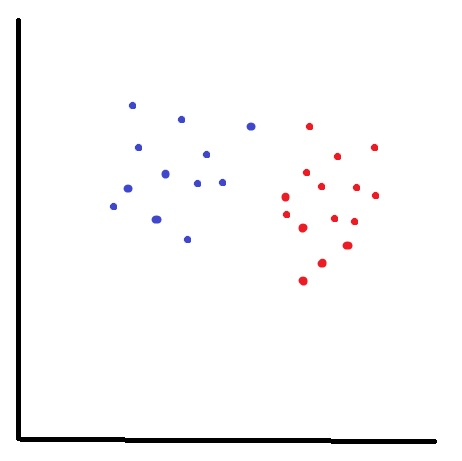What if I want to know about my new data point (green star)? Is it a 1 or a 0?I start by choosing a neighbor count – in this example I will choose 3, and I find the 3 nearest neighbors to my new point.

Let’s look at the results, I have 2 red(0) and 1 blue(1). Using basic probability, I am 67% (2/3) certain that you will not get in.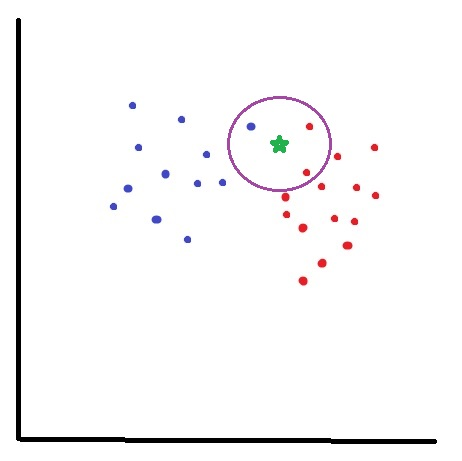## Now, let’s code it!

First we need to separate our data into 2 dataframes: Our training set X (Score) and our target set y (Accepted)

df.pop() removes the Accepted column from your dataframe and places it in a newly created one.### Import sklearn

sklearn is a massive library of machine learning algorithms available for Python. Today we are going to use KNeighborsClassfier

So below imported KNeighborsClassifier from sklearn.neighbors

Next I set my neighbor count to 5. You can experiment with other numbers and see how works out for you. Setting the neighbor count is something you kind of have to develop a feel for.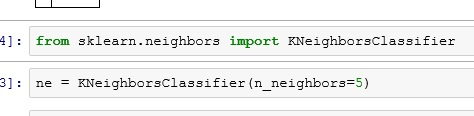Now let’s fit the model with our training set(X) and target set(y)Now we can use our model to make predictions.

ne.predict() will return 1 or 0 – (Accepted or Not)

while ne.predict_proba() will return a probability range. Results below read as (40% change of not Accepted(0), 60% chance of Accepted(1))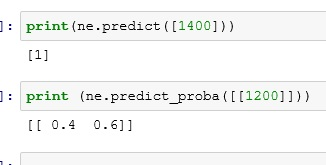So there you go, you have now built a prediction model using K Nearest Neighbor.

# Linear Regression using Excel

Link to video on Linear Regression using Excel

Regression Analysis is still the most popular method used in Predictive Analytics. The main reason is that it works. It is well known and understood. With its different flavors, regression analysis covers a width swath of problems. Another great reason to use it, is that regression tools are easy to find.

## What is Linear Regression?

Linear Regression is a method of statistical modeling where the value of a dependent variable based can be found calculated based on the value of one or more independent variables. The general idea, as seen in the picture below, is finding a line of best fit through the data. Using that line, you can then predict the value of Y given X.I am not going to go too deep into the math here. I highly the Khan Academy video posted below if you are looking to brush up on your statistics.

## Lets Start by Looking at the Data

If you download the Excel file at the top of the page, you will find 2 columns labeled Years and Salary. This example data set shows us the years of service and salary of 39 employees for an imaginary company.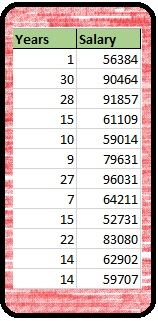What we are going to attempt to do is to develop a model using Linear Regression that will allow us to predict the salary of an employee given their years of service.

## Step 1: Build a Scatter Plot

The first thing we want to do is build a scatter plot. Excel makes this simple enough. Just highlight all of your data > select the Insert Tab from the Ribbon > Select Scatter from Charts: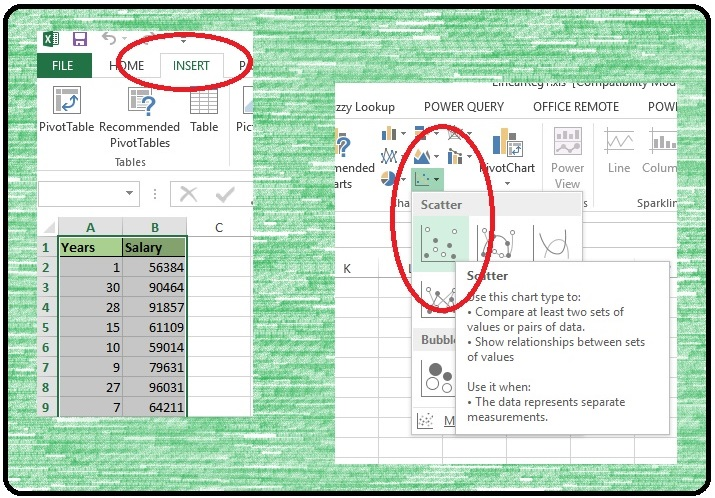What you will get should look something like this: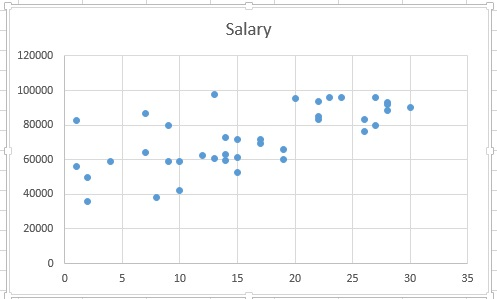We have a scatter chart with Salary on the Y Axis and Years on the X Axis. **Excel scatter charts set the left most column of the data set to the X Axis by default.

Before we move on, I want to take a moment to look at the scatter plot. Do you see a pattern? Can you see where you might be able to draw a line through the data?

I am not trying to just fill space here. I am asking a serious question. Because the answer is sometimes you will not see a pattern. Sometimes the scattering of data will be so random that there will no need to go forward with a linear regression. Learning to look for patterns in data visualizations is skill worth developing.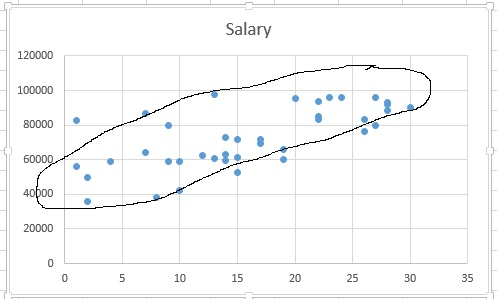In this example there is a general pattern, or more accurately, we see what looks like Positive Correlation. We call it positive because it appears that as X increases so does Y. So now that our scatter chart has passed the visual test, it is time perform our regression.

## Trend Line

Performing a simple linear regression in Excel is ridiculously easy. Simply click on your scatter plot > from the Ribbon select Chart Tools – Design > Add Chart Element > Trendline > LinearYour trendline appears on your chart. I personally find the line a little hard to see as is, so I am going to format it a bit.Start by double clicking on the trendline and the Format Trendline window will open on the right.

Line: — Color: Red  — Width: 3pt  — Dash type: Solid Line

Trendline Options — Select Display Equation on chart and Display R-squared value on chartAlright, that line is much easier to read. Now let us talk about the numbers in the circle. Now I know I said I was not going to get too deep into the math, but I feel I can’t do this subject justice without at least a cursory explanation of what is going on.What exactly did Excel do when it added the trendline? Technically it performed a statistical function known as Ordinary Least Squares.  What does that mean? Well if you wanted to attempt this by hand, one approach you could take would be to start by drawing a line that looked best to you. You would then measure the Residuals (the distance from the actual data points and line you drew)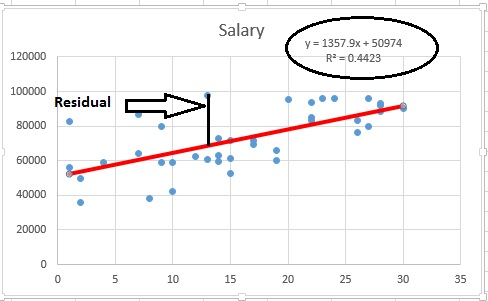You then repeat the process (picking a new line and measuring residuals) until you find the line that results in the lowest overall residual.Once you have it, you get the equation for your line:  y = 1357.9x+50974 (Luckily for us Excel makes the process a lot easier)

Now a quick refresher on the line formula: Y= mX + b (where m = Slope and b = Y-Intercept). This equation is what you would use to make predictions. In our equation a person with 0 years in service would have a salary of 50974: Y = 1357.9(0) + 50974 — Y= 50974. And each year of service would add 1357.90 to the salary.

Before we go start using your equation to start making predictions, we still need to discuss the R² you see below your line equation. I won’t bore you with how R² is calculated. You don’t really need to know how it is calculated to use linear regression, but you do need to know how to read it.

The simplest explanation I can give you for R² is that a value of 1 means perfect fit – every point in your data matches up to your line. 0 on the other hand, means your line doesn’t match anything. Our R² is 0.4423, which really is not that great. I generally prefer to aim for a R² value above 0.6.

How can we improve our R² value? My preference would be to get more data. We currently only have 39 tuples. More data could improve our accuracy. If more data is not available though, you can look at your outliers as Linear Regression can be greatly affected by outliers. Unfortunately outliers are often tricky to deal with. A person with 1 year of service making 100,000 a year would definitely be an outlier, but it is not an impossibility. If this employee is a highly experienced individual who just transferred from another company, it is totally feasible they could be earning 100,000.

The hard truth is, considering only the data we have, we cannot rightfully develop a reliable model. This happens more often than you might think. That is okay though, we will chalk this up as a learning experience and move on.

# Analytics: An Introduction

So exactly what is Analytics? Everyone is talking about it. Colleges and Universities are scrambling to develop programs in it. But what exactly does it mean?

## Definition

The the definition I like the best is this:

#### Analytics: Discovering and communicating meaningful patterns in data.

Analytics are traditionally broken down into the following catagories:

• Descriptive Analytics: Most people are familiar with this form. So familiar in fact, they probably do not refer to it as analytics. This is looking at past and current data to describe what is going on. Most standard business reporting falls into this category.
• Predictive Analytics: This is using available data to help predict future events or to provide best guess answers to fill in gaps in data. Using predictive analytics, you can predict how much a house will sell for or what items you should stock near the registers based on current conditions (example: Walmart discovered Pop-Tarts tend to sell well during hurricanes).
• Prescriptive Analytics: This is the cutting edge of analytics. Prescriptive analytics not only makes predictions about future events, but it utilizes decision making algorithms to determine how to respond to the events. Prescriptive analytics engines could, using the Pop Tarts example above, automatically reroute the shipment of Pop Tarts to stores in hurricane affected areas without any human intervention.

It should be noted that most companies today are still spending most of their time in the descriptive analytics world. That is not necessarily a bad thing. Being able to get the right information in front of a decision maker, in a format that is easily digestible, is a talent all within itself.

## Components

Analytics is not a 1 step process. It is actually a series of steps, often performed in an iterative manner. And just as each business problem is unique, so are the steps to the analytics process used to find the solution.

While the statement above is 100% percent true, I find it very unsatisfying. This is the kind of information I would find when I first developed an interest in analytics. So while I cannot give you a one size fits all answer, I feel that I at least owe you a better explanation than that.

For me, perhaps the best way to understand analytics, is to look at some of the more common tasks performed.

• Data Management: While designing, building, and maintaining databases and data warehouses may not typically fall under the responsibility of an analytics professional, having a general understanding of how they work is none the less important. Databases and data warehouses are where most businesses keep their data. If you want to be taken seriously as a data professional, you need to have a fundamental understanding of how data is stored and how to query the stored data. (Example Technologies: Hadoop, SQL Server, Oracle)
• Data Modeling: Data modeling is organizing data into logical structures so that is can be understood and manipulated by a machine. As a simple exercise, make a quick spreadsheet for sales amounts for  5 salespeople across 4 quarters. When you are done, look at the table you created. You have just modeled data. (Example Technologies: Excel, SQL Server, Oracle, Visio)
• Data Cleaning: While this may not be the sexiest part of the job, it is the part you will spend the most time on. 60-80% of your time will be spent in this phase of the job. And while there are some third party software applications out there that can help ease the pain (Alteryx comes immediately to mind), they are expensive and not every boss will be willing to spring for it. My suggestion is to put sometime aside to become very familiar with Excel. I do 90% of my data cleaning work in Excel and MS SQL Server. (Example Technologies: Excel, SQL Server, Oracle, Alteryx)
• Data Mining (Machine Learning): Now this is the cool stuff everyone is talking about. Data mining or machine learning, whichever you prefer to call it,  is the Artificial Intelligence (AI) portion of analytics. Data mining is difficult to provide a simple explanation for, but I will try anyway: In traditional programming, the programmer provides explicit instructions to the computer as to how to perform a task. With data mining, data sets are fed through an algorithm. The computer then determines the best way to solve the problem based on the data provided.

To help make this a little clearer, how about you try your hand at being the machine.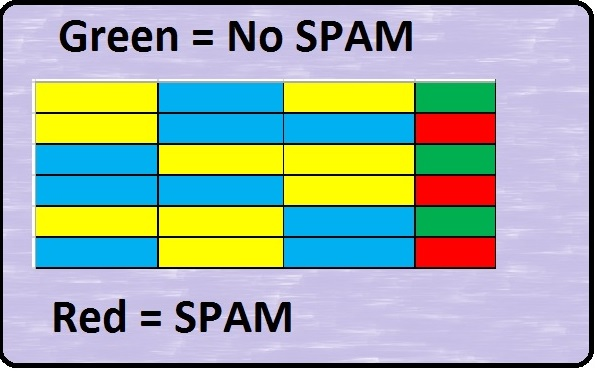Look at the pattern above. Without me providing you with any more information,                  you should be able to determine, that two blue squares in a row = SPAM. This is, at                 the most fundamental level, how data mining works. It pours over data and finds                   patterns. Knowing this pattern, if you were now shown only the first three columns               you would be able to predict whether the last column would be red or green.(Example Technologies: R, Python, SAS, XLMiner)

• Data Visualization: DataViz is fun. It is the real show stopper in the data world. Visualizations make the patterns pop off the page. There are a lot of great programs out there for data visualization. (Again, do not discount Excel — it has some great DataViz features). Now DataViz should rightfully be broken into two separate categories. The first is Exploratory. This is visualizations used by the data professional to help analyze and understand the data. The second is Production. This the finished product that ends up on reports and dashboards for the business users to see. (Example Technologies: Excel, Tableau, R, SAS)
• Optimization and Simulation: How often is there truly only one solution for a problem? Reality is sometimes the hardest part isn’t coming up with a solution to a problem, but deciding which solution to use. Building optimization models and running simulations helps to provide decision makers with quantitative data as to which solutions will be most effective. (Example Technologies: CPLEX, SAS, Solver)

## So I have to learn all of this…

That depends – If your goal to is be a Data Scientist, then yes, you need to learn everything mentioned above and then some (I hope you love Statistics). However, if you are a business user just trying to add analytic skill to your toolbox, my recommendation is to focus your efforts on becoming efficient in data cleaning. In the real world, when trying to put a report together, you often are given data from multiple sources and you have to cobble it together to make sense of it. Learning some data cleaning skills can save you hours on tasks like that.

Once you have workable data, take some time to learn some visualization techniques. An eye popping chart will always garner more attention than pages of numeric columns. Also, take a little time to learn some data mining skills. No one is expecting you to write the complex algorithms the PhD’s at Stanford and MIT are kicking out, but there actually are some pretty user friendly data mining programs out there that help you cull some real insight out of your data.

However you decide to go about it, Analytics is a fascinating, fast growing field. It truly is a 21st century skill. Here at Analytics4All.org, the philosophy is that everyone should develop some analytical talent. Computers were once the sole territory of the science geeks of the world and now they are in everyone’s pockets and purses. Analytics and data driven decision making should also be a accessible to all.